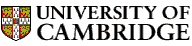Computer LaboratoryThe EDSAC Order CodeA n Add the number in storage location n into the accumulator. S n Subtract the number in storage location n into the accumulator. H n Transfer the number in storage location n into the multiplier register. V n Multiply the number in storage location n by the number in the multiplier register and add into the accumulator. N n Multiply the number in storage location n by the number in the multiplier register and subtract from the accumulator. T n Transfer the contents of the accumulator to storage location n and clear the accumulator. U n Transfer the contents of the accumulator to storage location n and do not clear the accumulator. C n Collate the number in storage location n with the number in the multiplier register, i.e. add a "1" into the accumulator in digital positions where both numbers have a "1", and a "0" in other digital positions. R 2 to the power (n-2) Shift the number in the accumulator n places to the right; i.e. multiply it by 2 to the power -n. L 2 to the power (n-2) Shift the number in the accumulator n places to the left; i.e. multiply it by 2 to the power n. E n If the number in the accumulator is greater than or equal to zero execute next the order which stands in storage location n; otherwise proceed serially. G n If the number in the accumulator is less than zero execute next the order which stands in storage location n; otherwise proceed serially. I n Read the next row of holes on the tape and place the resulting 5 digits in the least significant places of storage location n. O n Print the character now set up on the teleprinter and set up on the teleprinter the character represented by the five most significant digits in storage location n. F n Place the five digits which represent the character next to be printed by the teleprinter in the five most significant places of storage location n. X Round off the number in the accumulator to 16 binary digits. Y Round off the number in the accumulator to 34 binary digits. Z Stop the machine and ring the warning bell Explanatory Notes© 2004 University of Cambridge Computer Laboratory Please send any comments to pagemaster@cl.cam.ac.uk Page last updated on 11-Aug-2004 at 10:22 by Chris Hadley